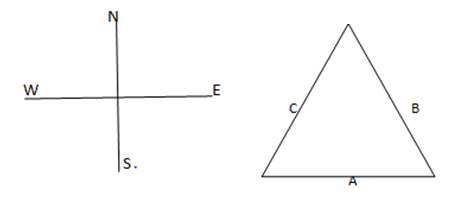# IAS Exam: IAS Prelims CSAT General Mental Ability Set 2

Here, we have provided the questions of General Mental Ability for the Civil Services CSAT Paper II of UPSC IAS Exam 2016. The pattern of these General Mental Ability Multiple Choice Questions has been kept in line with the questions asked in earlier attempts of Civil Services IAS Exam.In the Civil Services Prelim Examination, the CSAT paper has been made qualifying in nature from 2015 onwards. The candidates have to score at least qualifying marks in the paper but in case, if a candidate could not be able to score the required qualifying marks in CSAT Paper then his/her other papers will not be evaluated. So, it is important for the candidates to do preparation in such a manner that there must not lay them in any suspicion of underscoring.

Here, we have provided the questions of General Mental Ability for the Civil Services CSAT Paper II of UPSC IAS Exam 2016. The pattern of these General Mental Ability Multiple Choice Questions has been kept in line with the questions asked in earlier attempts of Civil Services IAS Exam.

Puzzle

1. In each of the questions given below which one of the five answer figures should come after the problem figures, if the sequence were continued: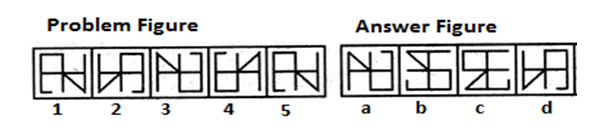Explanation:

The figure gets laterally inverted and vertically inverted alternately.

2. What among the following options can replace ‘?’ in the below sequence?
M M N M N O M N O P M N O P Q M N O P Q?

a) M
b) R
c) P
d) Q

Explanation:

(M), (MN), (MNO), (MNOP), (MNOPQ), (MNOPQR)

Category: Clock and Calendar

3. Kushal put his timepiece on the table in such a way that at 6 p.m. hour-hand points to North. In which direction the minute-hand will point at 9:15 p.m.?

a) South-East
b) South
c) North

d) west

Explanation: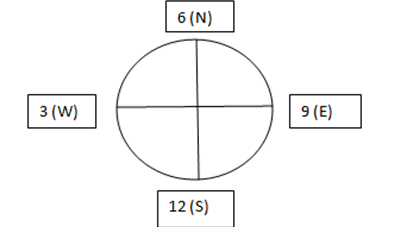The clock is placed such that west is at 3, north is at 6, east is at 9 and south is at 12. So, at 9:15 the minute hand will point to West direction.

4. What is the angle between the minute hand and the hour hand when the time is 1:40 PM?

a) 120
b) 170
c) 110
d) 130

Explanation:

The total angle made by the minute hand during an hour is 360o. If it takes 360o for an hour, it will take (40/60) * 360 = 240o. The angle between the hour hand the minute hand will therefore, be somewhere between 240 - 90 = 150o, as the hour hand is between 1 and 2.  The angle made by the hour hand when it moves from say 1 to 2 will be 30o. That is the hour hand makes 30o during the course of an hour.
The hour hand will therefore, move (40/60) * 30 = 20o.
Therefore, the net angle between the hour hand and the minute hand will be 150 - 20 = 130o.

5. Find the next term in the series: ACE, BDF, GIK, __, MOQ, NPR

a) TUV
b) LPR
c) HJL
d) PQR

Explanation:

The letters with odd position values are taken in alternate groups. i.e., ACEGI, KMOQS, UWYAC similarly the letters with even position values are taken in the other positions. I.e. DBFHJ, LNPRT, VXZBD. Hence, the missing group is LNPRT.

6. In a hostel, there are three options for students for evening classes : dancing, singing and swimming. Students must participate in at least one, and may participate in two or even in all three.  There are 240 students in the hostel.  There are 140 students in dancing, 146 in the singing, and 90 in the swimming.  Furthermore, 74 students are in both the dancing and singing, 40 are in both the dancing and the swimming, and 16 students are in all three groups.  Fifty students are just in the singing, not in anything else.   How many students participate in only the swimming?

a) 28
b) 30
c) 32
d) 27

Explanation: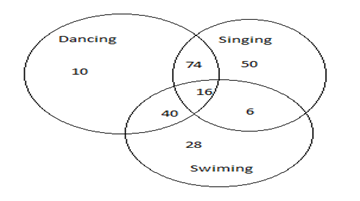7. Which one of the following Venn diagrams may represent the relationship among ‘metal’, ‘iron’ and ‘diamond’?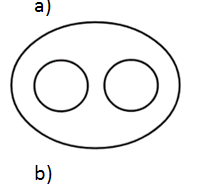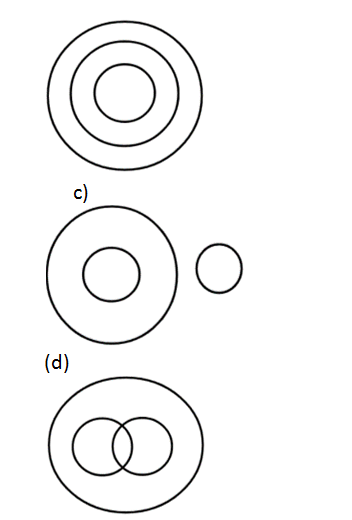8.    In a certain code language if “RAINBOW” is coded as “TCKPDQY”, then how will you code word “MATHEMATICS” in that language?

a) OCVKHPDVKEU
b) OCVJHPDWJDU
c) OCVJGOCVKEU
d) OCVKGOCVKEU

Explanation: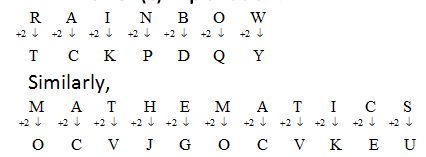So, the answer is option (c).

9. If A+C= D; C-A= B; A+D=5 & D-A=C, what is Z-X?

a) A
b) B
c) Y
d) C

Explanation:

All the alphabetical equations are with respect to   their positions in the alphabetic series. For, e. g, A (1) +C (3) = D (4) and so on. Thus Z (26)-X (24) = 2 which is B.

10. From the given equilateral triangle below, A, B and C run along the sides in clockwise direction and stop after covering 1 ½ sides. Which of the following statements is true?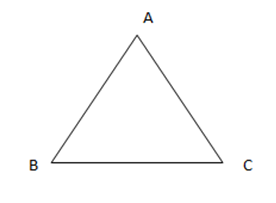a) B is to the West of C
b) A is to the south-west of B
c) B is to the north-west of A
d) C is to the north-east of A

Explanation:

The new positions are shown below: